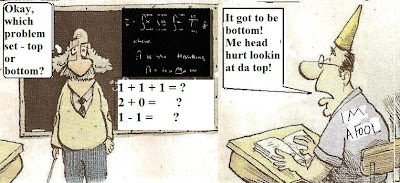## Friday, November 12, 2010

### Are American Students Really Math Dumpkopfs?The latest comparative math scores and analysis disclose American High Schoolers are literal goobers without a clue when it comes to the skills to do advanced math. Tests have disclosed the following dismal results of which we all ought to be collectively ashamed:

1) The U.S. placed 31st amongst 56 nations in demonstrating ability to perform advanced math skills (e.g. such as one would find in a typical intermediate algebra course, and trigonometry)

2) Only 6% of all U.S. High school students demonstrated a solid and consistent ability to solve such problems.

3) The state with the highest performance at advanced math level was Massachusetts, but even there barely 11% of high schoolers qualified.

4) White suburban kids in suburban schools aren't that great shakes either, with only 8% scoring at the advanced ability level.

Can readers meet this challenge? Here are some problems to see where you stand. Answers will be given in a blog next week:

In 1-3, simplify the given expression or perform the given operation then simplify as much as possible.

1) 5 (2x^2 - 6x)- (4x^2 - 3x)

2) 4^1/2 - 3^1/2

3) (x^2 + 2x - 3)/ (x^2 - 3x + 2)

In 4-5, factor completely:

4) x^2 - 9x + 18

5) x^2 + 10x + 25 - 9y^2

6) Graph the functions: f(x) = 2x and g(x) = 2x + 4 on the same coordinate axes.

7) Find the slope of any line that is perpendicular to the line whose equation is:

x + 4y - 8 = 0

8) Write the equation of the line passing through the point (3, -5) and perpendicular to the line whose equation is: x + 4y - 8 = 0